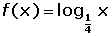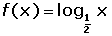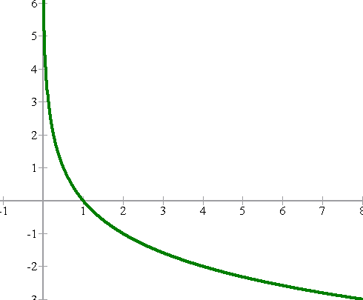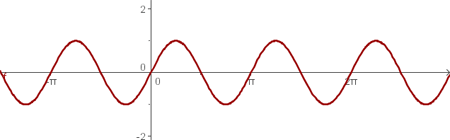## Exercise 1## Exercise 2## Exercise 3## Exercise 4f(x) = ln x

## Exercise 6## Exercise 7## Exercise 8## Exercise 9## Solution of exercise 1

Graph the exponential function:x f(x) = 3x −3 −2 −1 0 1 2 3 1/27 1/9 1/3 1 3 9 27## Solution of exercise 2

Graph the exponential function:x f(x) = (2/5)x −3 −2 −1 0 1 2 3 15.625 6.25 2.5 1 0.4 0.16 0.064## Solution of exercise 3

Graph the exponential function:x f(x) = (3/2)x −3 −2 −1 0 1 2 3 27/8 9/4 3/2 1 2/3 4/9 8/27## Solution of exercise 4

Graph the logarithmic function:x f(x) 1/8 1/4 1/2 1 2 4 8 −3 −2 −1 0 1 2 3## Solution of exercise 5

Graph the logarithmic function:

f(x) = ln x

 x f(x) 0.1 0.5 1 2 3 4 5 −2.3 −0.7 0 0.7 1.1 1.4 1.6## Solution of exercise 6

Graph the logarithmic function:x f(x) 1/8 1/4 1/2 1 2 4 8 3 2 1 0 −1 −2 −3## Solution of exercise 7

Graph the logarithmic function:x f(x) 1/8 1/4 1/2 1 2 4 8 3/2 1 1/2 0 −1/2 −1 −3/2## Solution of exercise 8

Graph the trigonometric function:x f(x) 0 π/4 π/2 3π/4 π 5π/4 3π/2 7π/4 2π 0 −0.7 −1 −0.7 0 0.7 1 0.7 0## Solution of exercise 9

Graph the trigonometric function:x f(x) 0 π/4 π/2 3π/4 π 5π/4 3π/2 7π/4 2π 0 1 0 −1 0 1 0 -1 0